Next: Streamlines and streamtubes Up: The equation of continuity Previous: The equation of continuity

## Incompressible flow

Many liquids are hard to compress (i.e., their volumes or densities don't change much when you squeeze them), so that the densityis essentially a constant. For such an incompressible fluid the equation of continuity simplifies to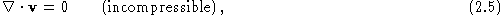so that the velocity fieldis a solenoidal vector. This condition is analogous to the condition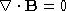which we encounter in electromagnetism--the magnetic field has zero divergence.

Incompressible flows are much easier to treat theoretically than compressible flows, so this is a very useful simplifying assumption. You might worry about applying it to gases, but it turns out that even there it often works well. In fact, if U is some characteristic flow velocity, and c is the speed of sound in the fluid, then the fluid can be treated as incompressible whenever. This is often expressed in terms of the Mach number Ma, defined as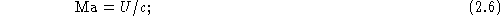then if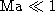the fluid can be treated as incompressible. For instance, the speed of sound in air is 330 m/s (740 mi/h); therefore, fluid flow around people, cars, trains, as well as fluid flow in hurricanes and tornadoes, all satisfy the incompressibility condition. Air flow around commercial airliners (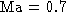) violates this condition, as does supersonic flight.

Vittorio Celli
Mon Aug 11 22:46:35 EDT 1997